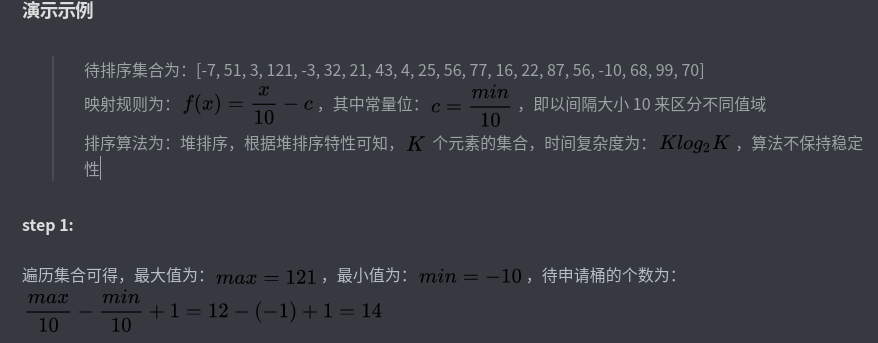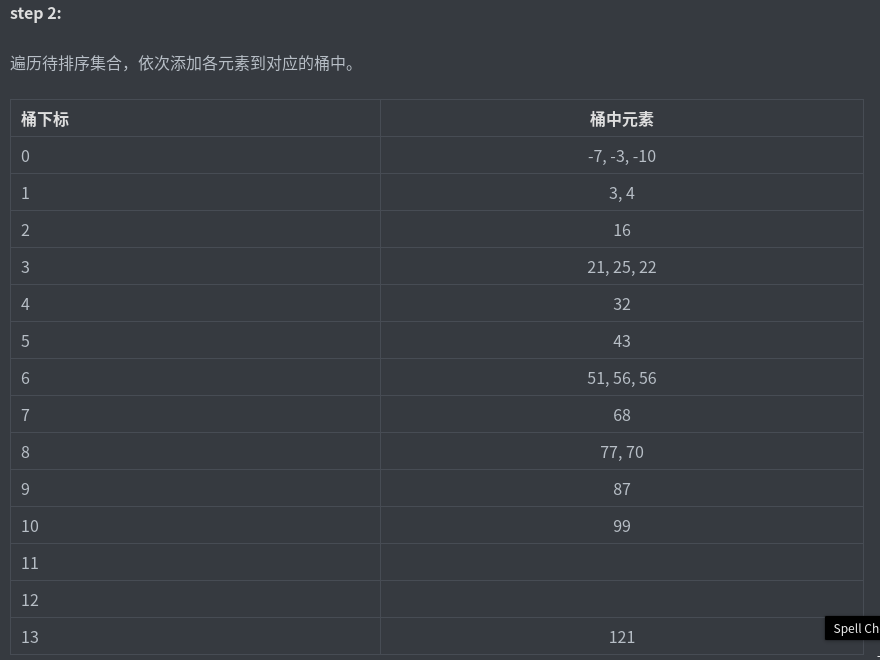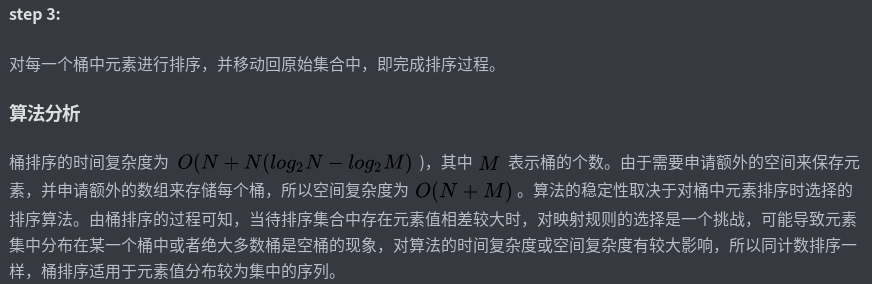# 排序系列计数 / 基数﻿

# 桶排序

﻿

﻿

﻿

• 元素值域的划分，也就是元素到桶的映射规则。映射规则需要根据待排序集合的元素分布特性进行选择，若规则设计的过于模糊、宽泛，则可能导致待排序集合中所有元素全部映射到一个桶上，则桶排序向比较性质排序算法演变。若映射规则设计的过于具体、严苛，则可能导致待排序集合中每一个元素值映射到一个桶上，则桶排序向计数排序方式演化。

• 排序算法的选择，从待排序集合中元素映射到各个桶上的过程，并不存在元素的比较和交换操作，在对各个桶中元素进行排序时，可以自主选择合适的排序算法，桶排序算法的复杂度和稳定性，都根据选择的排序算法不同而不同。

﻿

### 算法过程

﻿

1. 根据待排序集合中最大元素和最小元素的差值范围和映射规则，确定申请的桶个数；

2. 遍历待排序集合，将每一个元素移动到对应的桶中；

3. 对每一个桶中元素进行排序，并移动到已排序集合中。

﻿

﻿

### 演示示例﻿﻿﻿

﻿

﻿

# 计数排序

﻿

﻿

﻿

1. 申请一个ｋ+1的数组，用来存放排序元素的计数，ｋ可以理解为排序数组中的最大值，遍历整个待排序数组A，将A中每个元素对应C中的元素大小+1

2. 将C中每个i位置的元素大小改成C数组前i项和，这样就知道每个元素具体的位置了；

3. 再申请一个和原数组等长的新数组Ｂ，这样将C中的值恢复到Ｂ中，即完成排序；（恢复到Ａ中也是可以的，这里没有覆盖原数组，新申明了一个）

public static int[] countSort(int[] nums, int k) {        Arrays.nonBlank(nums);        int[] c = new int[k + 1];        int[] b = new int[nums.length];        for (int i = 0; i < nums.length; i++) {            c[nums[i]] += 1;        }        for (int i = 0; i < c.length - 1; i++) {            c[i + 1] = c[i] + c[i + 1];        }        for (int i = nums.length - 1; i >=0; i--) {            b[c[nums[i]] - 1] = nums[i];            c[nums[i]]--;        }        return b;    }

﻿

﻿

﻿

﻿

# 基数排序

﻿

static void count_sort(int a[], int size, int exp) {    int[] output = new int[size];             // 存储"被排序数据"的临时数组    int i;    int[] buckets = new int;    // 将数据出现的次数存储在buckets[]中    for (i = 0; i < size; i++)        buckets[(a[i] / exp) % 10]++;    // 更改buckets[i]。目的是让更改后的buckets[i]的值，是该数据在output[]中的位置。    for (i = 1; i < 10; i++)        buckets[i] += buckets[i - 1];    // 将数据存储到临时数组output[]中    for (i = size - 1; i >= 0; i--) {        output[buckets[(a[i] / exp) % 10] - 1] = a[i];        buckets[(a[i] / exp) % 10]--;    }    // 将排序好的数据赋值给a[]    for (i = 0; i < size; i++)        a[i] = output[i];}/*     * 基数排序     *     * 参数说明：     *     a -- 数组     *     size -- 数组长度     */static void radix_sort(int a[], int size) {    int max = get_max(a, size);    for (int exp = 1; max / exp > 0; exp *= 10)        count_sort(a, size, exp);}

﻿

# 总结

﻿

﻿

﻿## 评论